# Easiest colleges to get into in California

Top 10 colleges in California with the highest acceptance rates
What are the easiest colleges to get into in California? We've got you covered. We've compiled a national college database and have created a list of the easiest colleges to get into in California below. These are the easiest 4 year colleges to get into in California that have the highest acceptance rates, and are more likely to accept low GPAs, GEDs, and everything in between. There are tons of good colleges below that accept more applicants, and we also share their average SAT and ACT scores so that you can see where you're most competitive. Read on to find out more.

## Ashford University acceptance rate

Ashford University acceptance rate is 100%.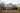The average SAT score for Ashford University is 970.

• SAT composite: 970
• SAT math: 485

The average ACT score for Ashford University is 18.

## California State University Bakersfield acceptance rate

California State University Bakersfield acceptance rate is 100%.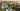The average SAT score for California State University Bakersfield is 993.

• SAT composite: 993
• SAT math: 490

The average ACT score for California State University Bakersfield is 19.

## Sonoma State University acceptance rate

Sonoma State University acceptance rate is 92%.The average SAT score for Sonoma State University is 1080.

• SAT composite: 1080
• SAT math: 530

The average ACT score for Sonoma State University is 22.

## Marymount California University acceptance rate

Marymount California University acceptance rate is 90.9%.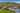The average SAT score for Marymount California University is 1071.

• SAT composite: 1071
• SAT math: 530

The average ACT score for Marymount California University is 21.

## Mills College acceptance rate

Mills College acceptance rate is 86.7%.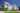The average SAT score for Mills College is 1230.

• SAT composite: 1230
• SAT math: 580

The average ACT score for Mills College is 26.

## California State University Stanislaus acceptance rate

California State University Stanislaus acceptance rate is 85.8%.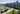The average SAT score for California State University Stanislaus is 1000.

• SAT composite: 1000
• SAT math: 490

The average ACT score for California State University Stanislaus is 19.

## Saint Mary's College acceptance rate

Saint Mary's College acceptance rate is 81.6%.The average SAT score for Saint Mary's College is 1160.

• SAT composite: 1160
• SAT math: 570

The average ACT score for Saint Mary's College is 25.

## Mount Saint Mary's College acceptance rate

Mount Saint Mary's College acceptance rate is 81%.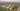The average SAT score for Mount Saint Mary's College is 1020.

• SAT composite: 1020
• SAT math: 500

The average ACT score for Mount Saint Mary's College is 18.

## Notre Dame de Namur University acceptance rate

Notre Dame de Namur University acceptance rate is 80.1%.The average SAT score for Notre Dame de Namur University is 1060.

• SAT composite: 1060
• SAT math: 520

The average ACT score for Notre Dame de Namur University is 21.

## California State University Channel Islands acceptance rate

California State University Channel Islands acceptance rate is 78%.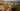The average SAT score for California State University Channel Islands is 1070.

• SAT composite: 1070
• SAT math: 530